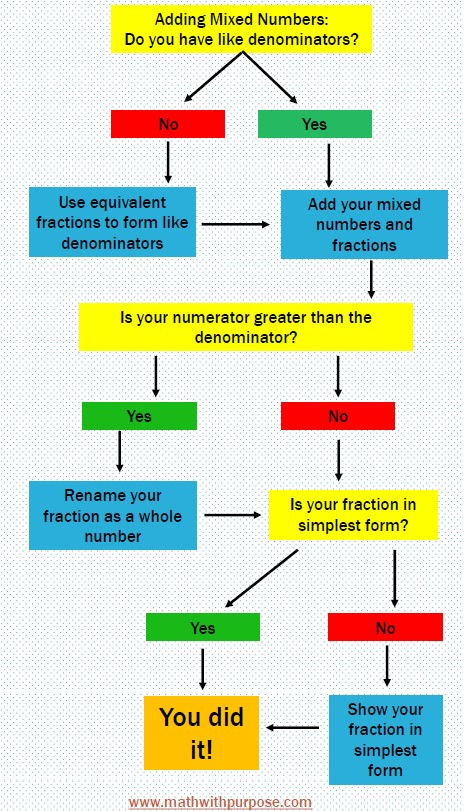top of page
Search
• Jessica Kaminski

# Flow Charts to Help Students Think Aloud in Math

In reading, we teach students how to think aloud. We read aloud to them and stop and model the questioning that might occur as a reader. We ask questions such as "Does that make sense to me?" or even "Do I know what's happening in the story?" From a young age, we model metacognition through the act of reading aloud and sharing our thinking.

As students begin to solve multi-step problems in math, we have to encourage students to do that same process. We need students to stop and ask themselves whether their answer makes sense or what they should do next. This begins in 2nd grade as students solve multi-digit addition and subtraction problems and even two-step word problems.

1. First he has to check if the denominators are the same.

2. Then, he has to add the numerators.

3. If the answer is greater than 1, he has to convert it to a mixed number.

4. Finally, he has to check that the answer is in simplest form.

So many steps and so many things to remember. How do we help our students to remember these processes and make sense of them at the same time?The first thing to remember is that students will not connect their learning unless they understand how it works. Most students can memorize something short term but may forget when they don't use it anymore. When they learn a concept by first experiencing it with a concrete experience, they will have a physical memory of working with the task. Then, students can represent the same concept with visuals and the abstract mathematical notation. This is so important with any mathematical concept, and you can find out more by looking at Jerome Bruner's concrete-pictorial-abstract theory.

Once students have learned the concepts, they begin to apply it to a process. Take our above example. Students learn that fractions greater than 1 can be represented by improper fractions and mixed numbers. They learn that they can be added by combining the whole numbers and the numerators of each fraction. They see that the denominator stays the same, because it is describing the whole. Then, students learn about equivalent fractions and how the mixed number can be renamed in its simplest form.Now we come full circle. Students have learned the concepts and are now applying them to a process with a TON of steps. How do we help our students to remember all these steps without teaching them to merely memorize procedures? Short Answer: We help them to think aloud their process by modeling metacognition. I find the best way to do this is to use a flow chart.

The flow chart above is one that students can use to follow and make decisions when adding mixed numbers. This flow chart helps students to work through the steps by making decisions and not just following a set of rules. They have to decide if they are ready for the next step by evaluating their work.

I think this would be a great way to help our students work through processes while still showing understanding. I would love for you to give it a try. Click below to grab your free download. Try it out and leave me a comment!